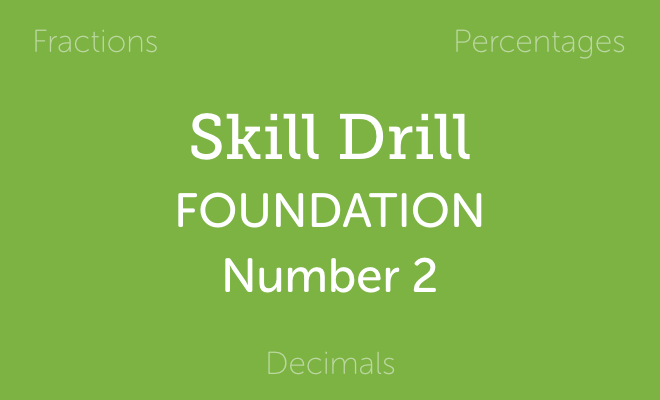Refer a friend and get % off! They'll get % off too.Foundation Number Skill Drill 2

This pack contains a series of 5 worksheets (with accompanying solutions) and a student 'skill tracker'.

Each worksheet includes 15 questions, one on each of the following topics:
1) Simplifying fractions
2) Converting a mixed number to an improper fraction
3) Converting an improper fraction to a mixed number
4) Fraction of an amount
5) Adding/subtracting fractions (same denominators)
6) Adding/subtracting fractions (different denominators)
7) Multiplying fractions
8) Dividing fractions
9) Percentage of an amount
10) Ordering decimals
11) Write a decimal as a percentage
12) Write a fraction as a decimal
13) Write a percentage as a fraction
14) Write a decimal as a fraction
15) Ordering a decimal, a fraction and a percentage

How could I use a Skill Drill?
That’s entirely up to you! Below are some suggestions though:
• Over a week or two to check progress as you ‘attack’ these topics in lessons
• As a diagnostic tool with a new group
• Set as a homework
• As a starter activity once a week to check skill retention after covering these
skills.

Who is this Skill Drill for?
This Skill Drill is primarily targeted at Foundation GCSE students aiming for a C. Although few of the included skills in this particular drill are ‘Grade C skills’ in and of themselves, they are still common weaknesses for such students.

Anything else I should know?
The included student tracker is ideal to allow students to track their own progress on each topic across the 5 drills.

You will get a PDF (5MB) file

£ 2.00

£ 2.00

Discount has been applied.

or